# 63 and Twelve Quick Clues

63 is a composite number. 63 = 1 x 63, 3 x 21, or 7 x 9. Factors of 63: 1, 3, 7, 9, 21, 63. Prime factorization: 63 = 3 x 3 x 7, which can also be written 63 = 3² x 7.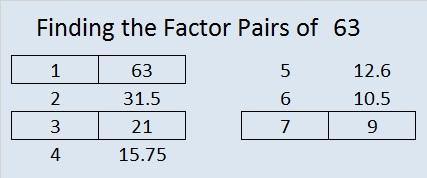Sometimes 63 is a clue in the FIND THE FACTORS puzzles. Even though it has other factors, the only multiplication fact we use is 7 x 9 = 63.All Previously Published Puzzles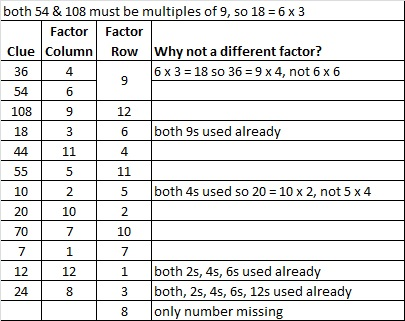Advertisements

# 25 and Level 3

25 is a composite number, and it is 5 squared. 25 = 1 x 25 or 5 x 5. Factors of 25: 1, 5, 25. Prime factorization: 25 = 5 x 5, which can also be written 25 = 5².

Since √25 = 5, a whole number, 25 is a perfect square.When 25 is a clue in the FIND THE FACTORS puzzles, write 5 in both the corresponding factor row and the factor column.Write the numbers 1-12 in the top row and again in the first column so that those numbers are the factors of the given clues. Use logic, not guessing and checking, to find its unique solution. Level 3 puzzles are designed to be solved starting from a row at the top of the puzzle with 2 clues. First find the common factor of those two clues that will allow you to write only numbers from 1 to 12 in the factor row at the top of the puzzle. Then factor row by row to the bottom of the puzzle making sure each number from 1 to 12 is written only once in both the factor row and the factor column. You will notice a rhythm for the answers as you work. Good luck!

This week’s puzzles are also available in an excel file here if you have a spreadsheet program on your computer. If you enable editing in excel, you can type your answers directly onto the puzzle, and you can also easily print the puzzles.

Here is the solution to last week’s level 3 puzzle:# 22 Factor Trees

22 is a composite number. 22 = 1 x 22 or 2 x 11. Factors of 22: 1, 2, 11, 22. Prime factorization: 22 = 2 x 11.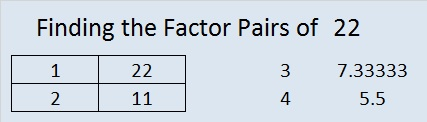When 22 is a clue in the FIND THE FACTORS 1 – 12 puzzles, use 2 and 11 as the factors.

‘Tis the season to use factor trees to find all the factors of a number. Here is a factor tree that shows all the prime factors of 560. Next to it is a factor tree for 324. Do you see all of their prime factors clearly?

Because sometimes one can’t “see the factors for the trees,” I recommend circling the prime factors or doing something else to make them more distinct.  Here are the trees again with every prime factor clearly visible:

I make logic puzzles based on the multiplication table. The puzzles for today’s post could also be called factor trees because you have to factor the clues to find the solution, and the puzzles are shaped like evergreen trees in whole or in part. I’ve even included a tree hanging from the ceiling, of all places! It may seem like a modern novelty, but people have been hanging Christmas trees upside down in Eastern Europe for centuries. To solve the puzzles either cut and paste the puzzles into a document to print or click 12 Factors 2013-12-12.

# 19 Last-Minute Gift

• 19 is a prime number.
• Prime factorization: 19 is prime.
• The exponent of prime number 19 is 1. Adding 1 to that exponent we get (1 + 1) = 2. Therefore 19 has exactly 2 factors.
• Factors of 19: 1, 19
• Factor pairs: 19 = 1 x 19
• 19 has no square factors that allow its square root to be simplified. √19 ≈ 4.3588989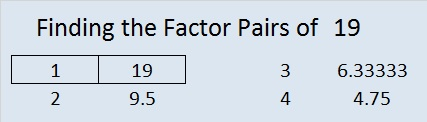How do we know that 19 is a prime number? If 19 were not a prime number, then it would be divisible by at least one prime number less than or equal to √19 ≈ 4.4. Since 19 cannot be divided evenly by 2 or 3, we know that 19 is a prime number.

19 is never a clue in the FIND THE FACTORS puzzles.

It’s Christmas Eve or even Christmas day, and maybe all of your shopping didn’t get done. Maybe you didn’t want to drive anyplace because of bad weather, or your favorite stores were closed early for the holiday. Well, if someone on your list likes number placing puzzles (like Sudoku or Kakuro), then I have a last-minute gift idea for you, and it’s free. I design a number placing logic puzzle based on the multiplication table called FIND THE FACTORS. If you have a computer, the internet, and a printer, you can print a little holiday booklet filled with these puzzles and give it as a gift. If the person on your gift list is many miles away, you can even send the booklet electronically. This last minute gift is good for the brain and can be good for the memory. The level 1 and level 2 puzzles can be solved by children 3rd grade and up, but most of the higher level puzzles will be challenging for everyone regardless of age.

Here is puzzle created to look a little like an angel just for the holidays: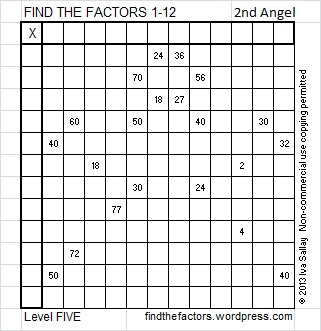To solve the puzzle above simply write the numbers 1 – 12 in the top row and also in the first column so that those numbers are the factors of the given clues. Okay, maybe it isn’t quite that simple. You have to know basic multiplication facts and use logic to figure out where the numbers go, and yes, I may try to trick you. But you and the people on your gift list have enough skills and persistence to find the one and only correct solution.

Now glancing at the puzzle above you may think you know all the answers, but…

This is what the solved puzzle looks like. Some of those factors may surprise you. That is why using logic is so important when solving the puzzles. (Once the factors are found, filling out the rest of the table is optional.)Click 2013 Factor Holiday to download a copy of the puzzle booklet. Some of the puzzles in the booklet are a little easier than the one above because they are a lower level or they only use factors up to 10. Have a very Merry Christmas and a Happy New Year!

Related articles with other ideas for last-minute gifts:

# 17 Christmas Angels

• 17 is a prime number.
• Prime factorization: 17 is prime.
• The exponent of prime number 17 is 1. Adding 1 to that exponent we get (1 + 1) = 2. Therefore 17 has exactly 2 factors.
• Factors of 17: 1, 17
• Factor pairs: 17 = 1 x 17
• 17 has no square factors that allow its square root to be simplified. √17 ≈ 4.123.How do we know that 17 is a prime number? If 17 were not a prime number, then it would be divisible by at least one prime number less than or equal to √17 ≈ 4.1. Since 17 cannot be divided evenly by 2 or 3, we know that 17 is a prime number.

17 is never a clue in the FIND THE FACTORS puzzles.

Many Christmas trees in the United States have been up and decorated for weeks. Some of them have a beautiful angel on the top to remind us of the angel that visited the shepherds. In Hungary, the angel is remembered in a different way. There the Christmas tree is put up on Christmas Eve. Tradition says that angels are the ones who decorate the tree with the delicious candies called szaloncukor. The candies are wrapped in specially prepared white tissue and fastened to the tree with white yarn. See the related articles at the end of the post for more information about this fascinating tradition.

The angel puzzles that I’ve made for this post have a few extra clues so they will be easier to solve. The first level 5 puzzle even has many of the same clues as the level 4 puzzle. Nevertheless, be careful because each level 5 angel has a few tricks up her sleeve. Still if you can write the numbers 1 to 12 in both the top row and the first column so that those numbers are the factors of the given clues, then you’ve solved the puzzle. There is only one solution to each puzzle. Click 12 Factors 2013-12-19 for a printable version of these and a few other puzzles.

Hungary:

United States:

# 14 Oh Christmas Tree

14 is a composite number. 14 = 1 x 14 or 2 x 7. Factors of 14: 1, 2, 7, 14. Prime factorization: 14 = 2 x 7.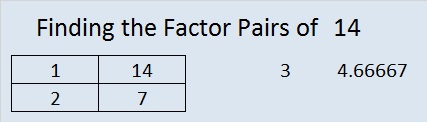When 14 is a clue in the FIND THE FACTORS  1 – 10 or 1 – 12 puzzles, use 2 and 7 as the factors.

O Christmas Tree, O Christmas Tree,

How lovely are your branches…

Do Christmas factor trees have lovely branches?  It depends on how they are constructed. For example here are 2 of the many possible factor trees for 1680. I think one of them is more lovely than the other.

This blog is actually about a logic puzzle that is based on the multiplication table. Today we have puzzles that look like Christmas trees, garland, lights, or blocks and a bright star for the very top.

Directions to solve the puzzles: In both the top row and the first column place the numbers 1 – 10 so that they are factors of the given clues. It may be more challenging than you think, especially for the higher level puzzles. If you click 10 Factors 2013-12-09, you can print the puzzles in color or black and white from an excel spreadsheet or you can type the answers directly on the spreadsheet. You must have a spreadsheet program on your device to access the file.

# 10 Just Like Sudoku?

10 is a composite number. 10 = 1 x 10 or 2 x 5. Factors of 10: 1, 2, 5, 10. Prime factorization: 10 = 2 x 5.When 10 is a clue in the FIND THE FACTORS puzzles, either 1 x 10 or else 2 x 5 will work for that particular puzzle.

On numerous occasions when I have demonstrated how to solve a Find the Factors puzzle, someone will remark that the puzzle is just like Sudoku. What are common factors that both a Find the Factors puzzle and a Sudoku puzzle will have? 1) Both will have only one solution. 2) Both require the solver to be able to count, write, and place the numerals 1 to 9, but Find the Factors also requires the number 10 be placed. 3) Both were originally designed to require logic to be solved. 4) Both puzzles utilize a square grid. 5) Both puzzles have several difficulty levels and variations that make the puzzles more challenging.

What factors do the two puzzles NOT have in common? 1) A difficult Sudoku puzzle can take some people almost an hour to solve while Find the Factors  would never take that long. 2) Sudoku has been a wildly popular puzzle while Find the Factors is known only among a small circle of people who have had some kind of contact with me. 3) Some more recent Sudoku puzzles require the solvers to guess and check which is getting away from its logic puzzle roots and is making it less popular for some people.  4) Sudoku could just as easily be made with letters of an alphabet, colors, or the names of the planets (if you include Pluto), while Find the Factors has to be made with numbers. 5) Sudoku requires only counting, while Find the Factors also requires the solver to factor and multiply. So really, if Find the Factors were just like Sudoku, it would look like this:Requiring skip counting to solve the Skipoku puzzle does make it more challenging, but I became annoyed with the skip counting by the time I finished the puzzle.

One complaint about some advanced Sudoku puzzles is the need to guess and check to find a solution. Is it necessary to guess and check all the possibilities to solve this level SIX Find the Factors puzzle?Click 10 Factors 2013-11-25 for more puzzles.

No. Guessing and checking is not necessary even though the clues 12 and 24 have several common factors.  We easily eliminate 1 and 2 because both of them would require a partner greater than 10. We also eliminate 12 because it is greater than 10. What about 3, 4, and 6? Do we have to try each of those possibilities? When we examine the puzzle we notice that it has 10 clues with only two of the clues paired together. We also notice there is one column that contains no clue, so supposedly any factor could fit there. Here is a chart of all the possible factors and the clues each could satisfy.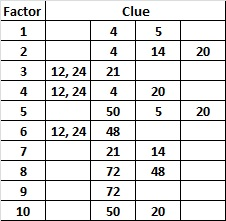Remember that each factor must be written twice, once in the factor row and one in the factor column. Notice that the number 9 is a factor of only one of the clues. That means that 9 has to be put over the column with no clues. From there it is easy to know where the other 9 goes and both 8’s and so forth until it is completed. Not all level SIX puzzles can be completed that easily, but using logic instead of guessing and checking is the key to solving these puzzles.